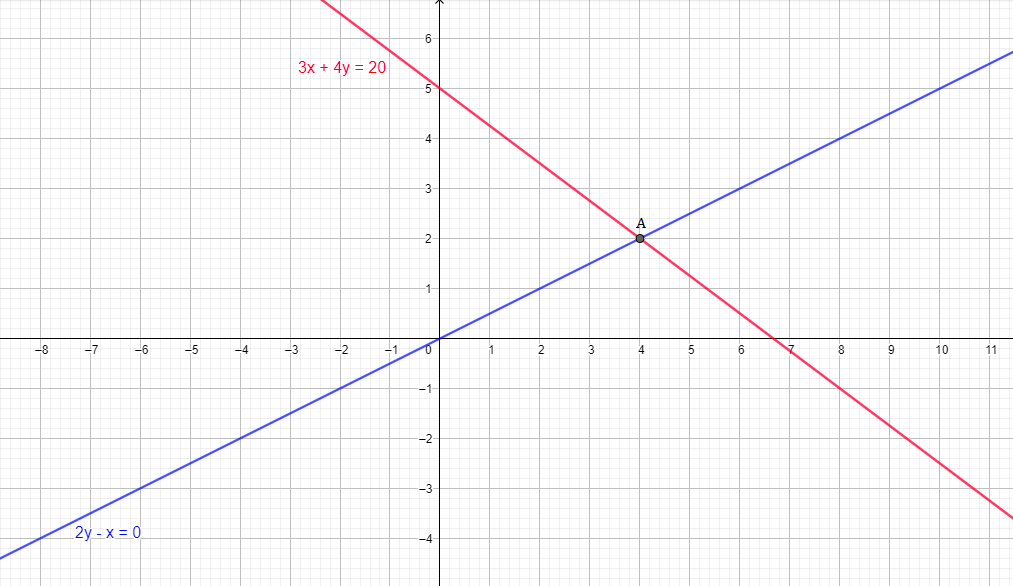# Akhila went to a fair in her village. She wanted to enjoy rides on the giant wheel and play hoopla (a game in which you throw a ring on the items kept in a stall and if the ring covers any object completely you get it ) if the number of times she played hoopla is half the number of times she rides the giant wheel if each ride cost Rs. 3 and a game of hoopla cost Rs. 4 and she spent Rs. 20 in the fair represent the situation algebraically and graphically.

Given:

Number of times she played hoopla is half the number of times she rides the giant wheel.

Cost of each ride = Rs. 3

Cost of a game of hoopla = Rs. 4

Total money Akhila spent = Rs. 20

To do: Here we have to represent the situation algebraically and graphically.

Solution:

Let the number of rides be  = $x$

Let number of game played = $y$

Now,

(Cost of each ride) $\times$ (No. of rides) $+$ (Cost of each game) $\times$ (No. of games) = Total money spent by Akhila

$3x\ +\ 4y\ =\ 20$   ....(i)

Number of times she played hoopla is half the number of times she rides the giant wheel:

$\frac{x}{2}\ =\ y$

$x\ =\ 2y$

$2y\ -\ x\ =\ 0$   ....(ii)

Using equation (i):

$3x\ +\ 4y\ =\ 20$

$x\ =\ \frac{20\ -\ 4y}{3}$

 x 0 4 y 4 2

$(x,\ y)$ = (0, 4) and (4, 2).

Using equation (ii):

$2y\ -\ x\ =\ 0$

$x\ =\ 2y$

 x 2 4 y 1 2

$(x,\ y)$ = (4, 2) and (2, 1)Solution of these two equations is where they intersect each other (point A). So,

$\mathbf{(x,\ y)}$ = (4, 2)

Therefore,

Number of rides be  = $x$ = 4

Number of game played = $y$ = 2

Updated on: 10-Oct-2022

33 Views